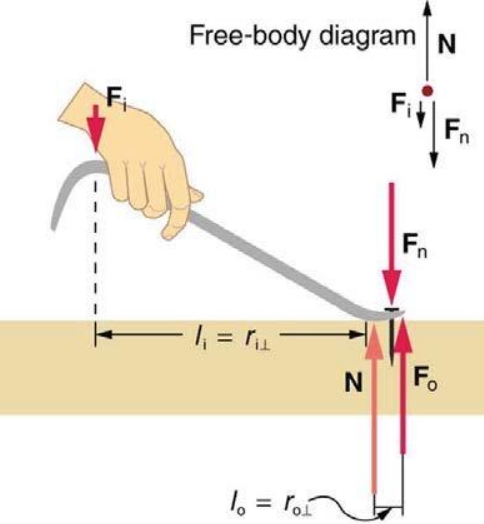# Problem: Consider the nail puller shown in the figure.(a) What is the mechanical advantage of a nail puller where you exert a force 46.5 cm from the pivot and the nail is 1.5 cm on the other side? MA =   (b) what minimum force must you exert to apply a force of 1050 N to the nail?

###### FREE Expert Solution

Part (a)

Mechanical advantage is the ratio of the distance input and the distance output.

MA = li/lo

100% (69 ratings)###### Problem Details

Consider the nail puller shown in the figure.

(a) What is the mechanical advantage of a nail puller where you exert a force 46.5 cm from the pivot and the nail is 1.5 cm on the other side?

MA =

(b) what minimum force must you exert to apply a force of 1050 N to the nail?